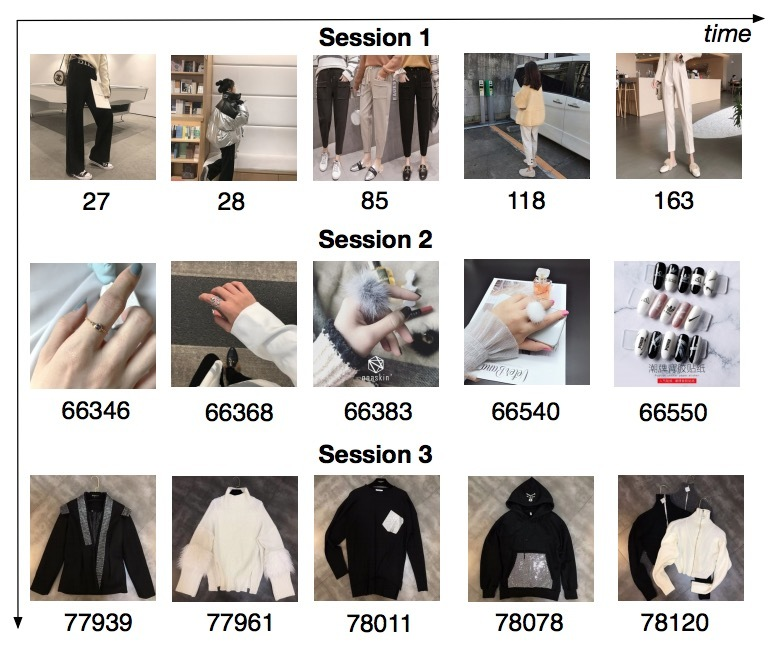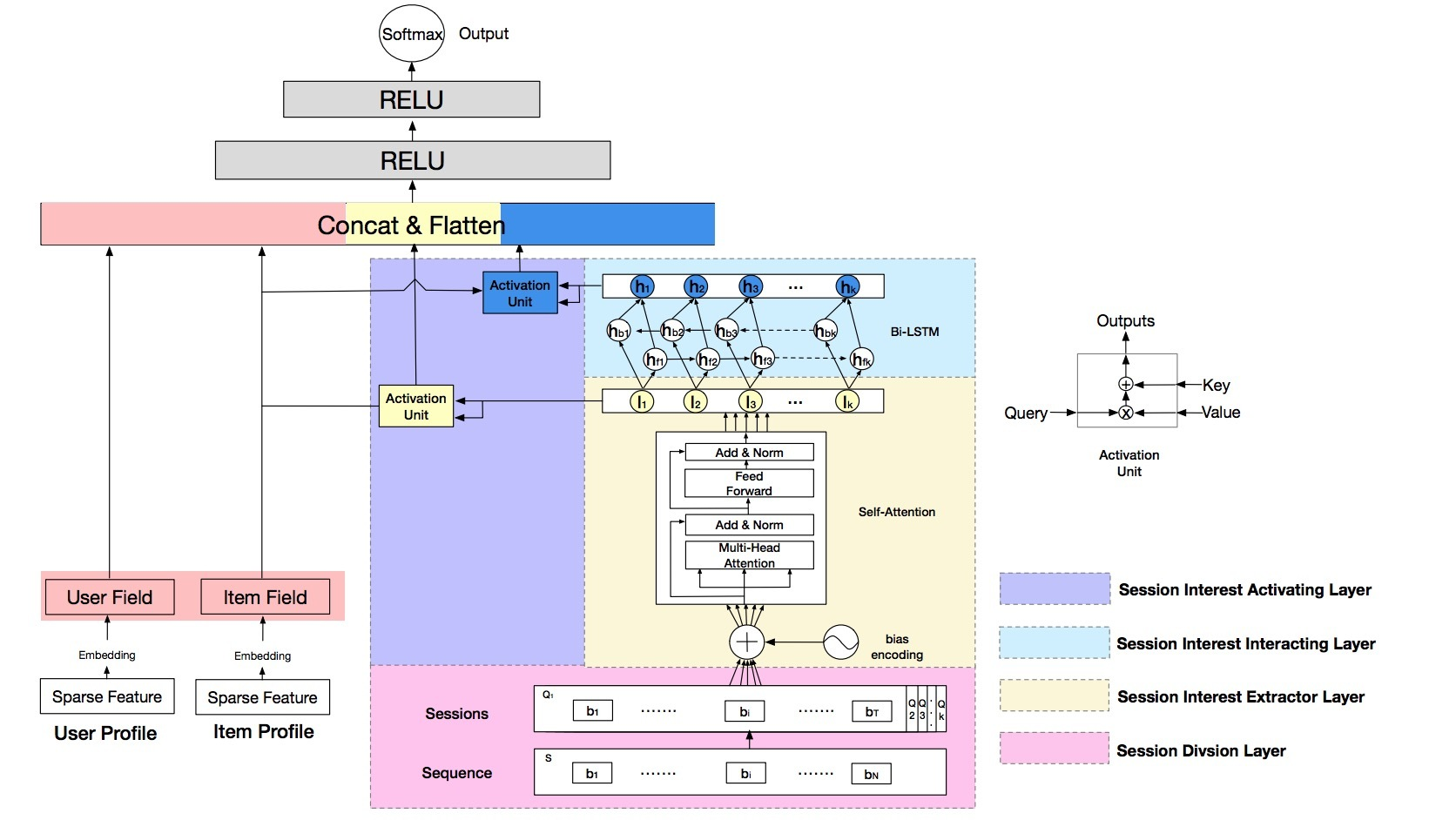# 推荐系统fairness

Alex Beutel等人在KDD 2019《Fairness in Recommendation Ranking through Pairwise Comparisons》中提出pairwise比较来看fairness。具体方法如下：

# 介绍

• Pairwise Fairness：
• Pairwise Regularization：
• 真实实验：

# 2.相关工作

Recommender System Fairness。在ranking和recommendation上的fairness上，已经有一些研究，但这些研究都从不同的视角出发。Zehlike从排序公平性的目标出发，但没有考虑推荐系统（它的数据是很稀疏的）。相似的，Singh采用一个full-ranking的公平性视角，可以通过一个后处理算法(post-processing) 对模型预测应用于推荐系统；接着将它移到模型训练中。所有这些工作[52,44,45,11]关注于一个非个性化的信息检索系统，其中主要关注每个item的相关labels；我们关于的个性化推荐场景存在：数据稀疏性和biases。[8,49]则关注于CF pointwise accuracy跨不同groups的差异，但没有将这些metrics连接到最终的rankings上。

Fairness Optimization. 许多方法的提出是为了解决公平性问题。Post-processing可以提供优雅解法【23，44】，但通常需要已经对于所有样本的group memberships，这对于demographic数据来说几乎是未知的。然而，许多方法在分类训练期间来优化fairness metrics，比如：constriaint-based optimization、adversarial learning、以及通过模型预测的regularzation。我们构建了这些regularization方法来提升我们推荐系统的fairness属性。

# 3.推荐的pairwise fairness

ranker是一个模型$f_{\theta}$，参数为$\theta$；该模型被训练来预测用户参与度$f_{\theta}(q,v) = (\hat{y},\hat{z}) \approx (y,z)$。最终，一个items的ranking会通过打分函数$g(\hat{y}, \hat{z})$来生成，用户会从由g排序的相关items R中选取topK个items。

…(1)

…(3) …(4)

# 参考

Minmin Chen、Alex Beutel等在《Top-K Off-Policy Correction for a REINFORCE Recommender System》中提出使用强化学习来提升youtube推荐。主要是从bias/variance的角度出发，具体方法如下：

# 摘要

• 1.将REINFORCE扩展到生产环境推荐系统上，动作空间有数百万；
• 2.使用off-policy correction来解决在从多种行为策略中收集的日志反馈的数据偏差（data biases）
• 3.提出了一种新的top-K off-policy correction来解释我们一次推荐多个items的策略推荐
• 4.展示了探索(exploration)的价值

# 1.介绍

• 2.Off-Policy候选生成：我们使用off-policy correction来从日志反馈中学习，这些日志从之前的model policies的一个ensemble中收集而来。我们会结合一个已经学到的关于行为策略(behavior policies)的神经网络模型来纠正数据偏差。
• 3.Top-K Off-policy Correction：我们提供了一个新的top-K off-policy correction来说明：我们的推荐一次输出多个items。
• 4.真实环境的提升：我们展示了在真实环境中（在RL文献中很少有这种情况），这些方法对于提升用户长期满意度的价值。

# 3.增强推荐• S：用于描述用户状态(user states)的一个连续状态空间（state space）
• A：一个离散的动作空间(action space)，它包含了推荐可提供的items
• $P: S \times A \times S \rightarrow R$：是一个状态转移概率
• $R: S \times A \rightarrow R$：回报函数(reward function)，其中$r(s,a)$是立即回报(immediate reward)，它通过在用户状态（user state）s上执行动作a得到
• $\rho_0$：初始state分布
• $\gamma$：对于future rewards的discount factor…(1)

…(2)

# 4.off-policy collrection

…(3)

Achiam证明了：该一阶近似对于学到的policy上的总回报的影响，会通过$O(E_{s \sim d^{\beta}} [D_{TV}(\pi \mid \beta)[s]])$来限定幅值，其中$D_{TV}$是在$\pi(\cdot \mid s)$$\beta(\cdot \mid s)$间的总方差，$d^{\beta}$是在$\beta$下的discounted future state分布。该estimator会权衡精确的off-policy correction的方差，并仍能为一个non-corrected policy gradient收集大的偏差，这更适合on-policy learning。

## 4.1 对policy $\pi_{\theta}$进行参数化

• user state （$s_t \in R^n$）: 我们对每个时间t上的user state建模，这可以同时捕获用户兴趣的演进，它使用n维向量$s_t \in R^n$来表示。
• action （$u_{a_t} \in R^m$）: 沿着该轨迹（trajectory）每个时间t上的action会使用一个m维向量$u_{a_t} \in R^m$进行嵌入。

…(4)

• $z_t \in R^n$是update gate
• $i_t \in R^n$是input gate

…(5)

• $v_a \in R^n$是每个action a在action space A中的另一个embedding（注：前面的$u_{a_t} \in R^m$是m维，而$v_a$是与$s_t$相同维度）
• T是时序(通常设置为1)。T值越大会在action space上产生一个更平滑的policy。

• 两个action embeddings$U \in R^{m \times \mid A \mid}$$V \in R^{n \times \mid A \mid}$
• 在RNN cell中的权重矩阵：$U_z, U_i \in R^{n \times n}, W_u, W_i, W_a \in R^{n \times m}$
• biases：$b_u, b_i \in R^n$## 4.2 估计behavior policy $\beta$

• (1) 在我们的系统中有许多agents，许多是不可控的
• (2) 一些agents具有一个deterministic policy，将$\beta$设置成0或1并不是使用这些日志反馈(logged feedback)的最有效方式

• (1) main policy $\pi_{\theta}$会使用一个weighted softmax来考虑长期回报(long term reward)进行有效训练；而behavior policy head $\beta_{\theta'}$只会使用state-action pairs进行训练（言下之意，不考虑reward?）
• (2) main policy head $\pi_\theta$只使用在该trajectory上具有非零回报（non-zero reward）的items进行训练(注3)；而behavior policy $\beta_{\theta'}$使用在该轨迹上的所有items进行训练，从而避免引入在$\beta$估计时的bias

## 4.3 Top-K off-policy Correction

• $\alpha_{\theta} (a \mid s) = 1 - (1- \pi_{\theta}(a \mid s))^K$：表示的是一个item a出现在最终的无重复集合A中的概率。这里，$K = \mid A'\mid >\mid A\mid = k$。（注：作为有放回(replacement)和去重（de-duplicate）抽样的结果，最终集合A的size是可变的）

…(6)

…(7)

• 随着$\pi_{\theta}(a\mid s) \rightarrow 0, \lambda_K(s,a) \rightarrow K$。对比起标准的off-policy correction，top-K off-policy correction会通过一个K因子来增加policy update；
• 随着$\pi_{\theta}(a \mid s) \rightarrow 1, \lambda_K(s,a) \rightarrow 0$。该乘子会使policy update归0
• 随着K的增加，以及$\pi_{\theta}(a \mid s)$会达到一个合理的范围, 该乘子会更快地将graident减小于0

## 4.4 Variance Reduction技术

Weight Capping。

…(8)

c的值越小，会减小在梯度估计时的方差，但会引入更大的bias。

NIS(归一化重要性抽样：Normalized Importance Sampling)

TRPO(Trusted Region Policy Optimization). TRPO会阻止new policy $\pi$背离behavior policy，它通过增加一个正则项来惩罚这两个policies的KL散度。它会达到与weight capping相似的效果。

# 6.实验结果

## 6.1 仿真

### 6.1.1 off-policy correction1
2
3
4
5
6
7
8
actions = [1,2,3,4,5,6,7,8,9,10]
b = lambda x: (11-x)/55.0
beta = [b(i) for i in actions]
rxb = [(i+1)*j for i, j in enumerate(beta)]
total = sum(rxb)
pi = [i/total for i in rxb]



### 6.1.2 Top-K-policy correction## 6.2 真实环境

### 6.2.2 Off-policy Correction

【注6】：实际中，我们使用一个相当小比例的用户做为test polulation；作为结果，我们记录的feedback则几乎通过control model获取actions数目

Capping

# PPO(openai)介绍

openai在《Proximal Policy Optimization Algorithms》提出了PPO。我们来看下：

# 2.背景: Policy Optimization

…(1)

• $\pi_{\theta}$是一个stochastic policy
• $\hat{A}$是一个在timestep t时advatage function的estimator
• 期望$\hat{E}_t[\cdots]$表示在一个会在sampling和optimization间做交替的算法中，一个有限batch的样本上的经验平均(empirical average)。

…(2)

## 2.2 Trust Region方法

…(4)

TRPO的理论证明建议我们使用一个正则项(penalty)来替代constraint，比如，对一些系数$\beta$求解以下没有constraint的最优化问题：

…(5)

# 3.对Surrogate Objective进行裁减（Clip）

…(6)

…(7)• 1.使用一些minibatch SGD的epochs，来优化KL-penalized objective：$L^{KLPEN}(\theta) = \hat{E}_t [\frac{\pi_{\theta}(a_t \mid s_t)}{\pi_{\theta_{old}}(a_t \mid s_t)} \hat{A}_t - \beta KL [\pi_{\theta_{old}} (\cdot \mid s_t), \pi_{\theta}(\cdot \mid s_t)] ]$
• 计算 $d = \hat{E}_t[KL[\pi_{\theta}(\cdot \mid s_t), \pi_{\theta}(\cdot \mid s_t)]]$
• if $% $
• if $d > d_{targ} \times 1.5, \beta \leftarrow \beta \times 2$

# 5.算法

….(9)

• $c_1, c_2$是系数
• S表示一个entropy bonus
• $L_t^{VF}$是一个squared-error loss：$(V_{\theta}(s_t) - V_t^{targ})^2$

…(10)

….(11)(12)# PPO(deepmind版)介绍

deepmind在《Emergence of Locomotion Behaviours in Rich Environments》提出了PPO。我们来看下：

# 2.使用Distributed PPO进行大规模增强学习

Policy gradient算法为连续控制（continuous control）提供了一个吸引人的范式。他们通过根据stochastic policy $\pi_{\theta}(a \mid s)$的参数$\theta$直接最大化rewards的期望和：

$\theta$对应的目标函数的梯度是：

• $b_t$是一个baseline（它不依赖于$a_t$或者future states和actions）。该baseline通常会选择$b_t = V^{\theta} (s_t) = E_{\theta} [R_t \mid s_t]$

Policy gradient的estimates可以有很高的variance，算法对于超参数的设置很敏感。有许多方法可以使得policy gradient算法更健壮。一种有效的measure是：采用一个trust region constraint，可以限制policy update的任意更新量(amount)。采用该思想的一种流行算法是：TRPO（trust region policy optimization）。在每个迭代中，给定当前参数$\theta_{old}$，TRPO会收集一个（相对较大）的batch数据，并优化surrogate loss：

$A^{\theta}$是advantage function，可以通过$A^{\theta}(s_t, a_t) = E_{\theta}[R_t \mid s_t, a_t] - V^{\theta}(s_t)$得到。

PPO算法可以被看成是一个依赖于一阶梯度的TRPO的近似版本，它可以更方便地使用RNNs，并可以用在一个大规模分布式setting中。trust region constraint通过一个正则项来实现。使用的正则项系数依赖于该是否先前违反了constraint。算法1展示了PPO算法的伪代码。John Schulman的公开提供的PPO实现对于核心算法增加了一些修改。它们包含了对inputs、rewards、以及在loss中的一个额外项（它会惩罚对于较大违反trust region constraint的情况）的normalization。我们采用在分布式setting中相似的augmentations，但发现在跨多个workers间对多个统计进行sharing和synchronization需要一些注意。我们的分布式实现(DPPO)采用tensorflow，参数在一个parameter server中，workers会在每个gradient step后同步它们的参数。伪码和详情在附件中提供。

## A.DPPO

A.1 算法详情normalization

• 1.我们会通过减mean并除以标准差，将observations（或者 states $s_t$）进行归一化。。。
• 2.我们会通过一个正在运行的关于标准差的estimate对reward进行缩放（scale），在整个实验过程中进行聚合

# 1.• 我们强调用户行为在每个session中高度同质，不同sessions差异很大。
• 设计了一个self-attention network以及bias encoding来获得每个session的精准兴趣表示。接着我们使用Bi-LSTM来捕获历史sessions间的顺序关系(sequential relationship)。最后，考虑到不同session interest在target item上的影响，我们使用local activation unit来聚合。
• 两组比较实验。表明DSIN效果更好。

# 2.相关工作

## 2.2 Session-based推荐

session的概率常被序列化推荐提及，但很少出现在CTR预测任务中。Session-based推荐受益于用户兴趣在sessions上动态演化的启发。GFF使用关于items的sum pooling来表示一个session。每个item具有两种表示，一个表示自身，另一个表示session的上下文(context)。最近，RNN-based方法被应用于session-based推荐中来捕获在一个session中的顺序关系。基于此，Li 2017提出了一个新的NARM（attentive neural networks framework）来建模用户的序列化行为，并能捕获用户在当前session中的主要目的。Quadrana 2017提出的Hierarchical RNN依赖于RNNs的latent hidden states跨用户历史sessions的演化。另外，Liu 2018 的RNNs使用self-attention based模型来有效捕获一个session的long-term和short-term兴趣。Tang 2018使用CNN、Chen 2018使用user memory network来增强序列模型的表现力。

# 3.DSIN

## 3.1 BaseModel

CTR预测任务中统计了大量信息特征。总共使用了三大组：User profile、item profile、User Behavior。每组包含了一些稀疏特征：

• User Profile包含了gender、city等；
• Item Profile包含了：seller id、brand id等；
• User Behavior包含了用户最近点击的item ids等

Embedding

MLP

Loss Function

## 3.2 模型总览• 1) session division layer，会将用户行为序列划分为sessions
• 2) session interest extractor layer：会抽取用户的session interests
• 3) session interest interacting layer：会捕获session interests间的顺序关系
• 4) session interest activating layer：会对与target item有关的session interests使用local activation unit

### Session Interest Extractor Layer

Bias Encoding

…(2)

• $w^K \in R^K$：是session的bias vector
• k：是sessions的索引
• $w^T \in R^T$：是在session中position的bias vector
• t：是在sessions中行为的索引
• $w^C \in R^{d_{model}}$：是在behavior embedding中unit position的bias vector
• c：是在behavior embedding中unit的index。

…(3)

Multi-head Self-attention.。在推荐系统中，用户的点击行为受许多因素（颜色、风格、价格等）的影响。Multi-head self-attention可以捕获不同表示子空间的表示。数学上，假设：$Q_k = [Q_{k1}; \cdots; Q_{kh}; \cdots; Q_{kH}]$，其中$Q_{kh} \in R^{T \times d_h}$$Q_k$的第h个head，H是heads的数目，$d_h = \frac{1}{h} d_{model}$$head_h$的输出如下计算：

…(4)

…(5)

…(6)

…(7)

…(8)

…(9)

…(10)